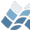NV5 GEOSPATIAL SOFTWARECAT_IDLARROW

## Name

CAT_IDLARROW
PURPOSE: Draw a vector(s) with an arrow head
CATEGORY: Graphics

## Calling Sequence

CAT_IDLARROW, x0, y0, x1, y1

## Inputs

(x0, y0) = coordinates of beginning of vector(s). May be arrays
or scalars. Coordinates are in DEVICE coordinates
unless otherwise specified.
(x1, y1) = coordinates of endpoint (head) of vector.
x0, y0, x1, y1 must all have the same number of elements.

## Keyword Parameters

DATA - if set, implies that coordinates are in data coords.
NORMALIZED - if set, coordinates are specified in normalized coords.
HSIZE = size of arrowhead. Default = 1/64th the width of the device,
(!D.X_SIZE / 64.).
If the size is positive, it is assumed to be in device
coordinate units. If it is NEGATIVE, then the head length
is set to the vector length * abs(hsize), giving heads
proportional in size to the bodies. The size is defined as
the length of each of the lines (separated by 60 degrees)
COLOR = drawing color. Default = highest color index.
HTHICK = thickness of heads. Default = 1.0.
SOLID = if set, make a solid arrow, using polygon fills, looks better
for thick arrows.
THICK = thickness of body. Default = 1.0.

## Outputs

No explicit outputs.

## Procedure

Straightforward.

## Examples

Draw an arrow from (100,150) to (300,350) in DEVICE units.
CAT_IDLARROW, 100, 150, 300, 350
Draw a sine wave with arrows from the line Y=0 to
sin(x/4).
X = FINDGEN(50)
Y = SIN(x/4) ;Make sin wave
PLOT, X, Y
CAT_IDLARROW, X, REPLICATE(0,50), X, Y, /DATA

## Modification History

DMS, Feb, 1992.